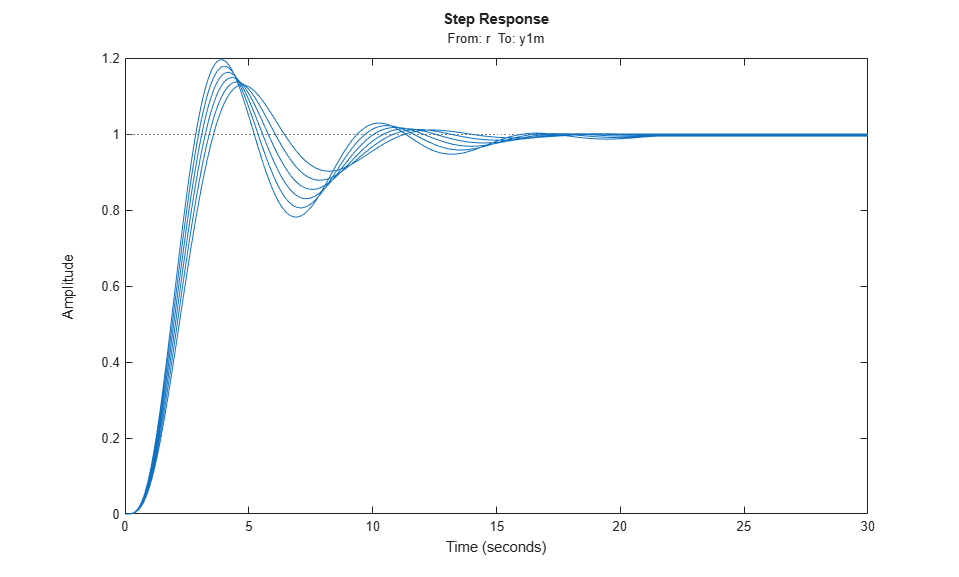Batch Linearize Model for Parameter Variations at Single Operating Point

In this example, you vary model parameters and linearize a model at its nominal operating conditions using the linearize command.

You can batch linearize a model for parameter variations at a single operating point to study:

• Plant dynamics for varying component tolerances.

• Controller robustness to variations in plant parameters.

• Transient responses for varying controller gains.

The scdcascade model contains two cascaded feedback control loops. Each loop includes a PI controller. The plant models, G1 and G2, are LTI models.

For this model, the model operating point represents the nominal operating conditions of the system. Therefore, you do not have to trim the model before linearization. If your application includes parameter variations that affect the operating point of the model, you must first batch trim the model for the parameter variations. Then, you can linearize the model at the trimmed operating points. For more information, see Batch Linearize Model at Multiple Operating Points Derived from Parameter Variations.

To examine the effects of varying the outer-loop controller gains, linearize the model at the nominal operating point for each combination of gain values.

Open the model.

open_system(sys)Define linearization input and output points for computing the closed-loop input/output response of the system.

io(1), the signal originating at the outport of the setpoint block, is the reference input. io(2), the signal originating at the outport of the Sum block, is the system output.

To extract multiple open-loop and closed-loop transfer functions from the same model, batch linearize the system using an slLinearizer interface. For more information, see Vary Parameter Values and Obtain Multiple Transfer Functions.

Vary the outer-loop controller gains, Kp1 and Ki1, within 20% of their nominal values.

Kp1_range = linspace(Kp1*0.8,Kp1*1.2,6);
Ki1_range = linspace(Ki1*0.8,Ki1*1.2,4);
[Kp1_grid,Ki1_grid] = ndgrid(Kp1_range,Ki1_range);

Create a parameter structure with fields Name and Value. Name indicates which the variable to vary in the model workspace, the MATLAB® workspace, or a data dictionary.

params(1).Name = 'Kp1';
params(1).Value = Kp1_grid;
params(2).Name = 'Ki1';
params(2).Value = Ki1_grid;

params is a 6-by-4 parameter value grid, where each grid point corresponds to a unique combination of Kp1 and Ki1 values.

Obtain the closed-loop transfer function from the reference input to the plant output for the specified parameter values. If you do not specify an operating point, linearize uses the current model operating point.

G = linearize(sys,io,params);

G is a 6-by-4 array of linearized models. Each entry in the array contains a linearization for the corresponding parameter combination in params. For example, G(:,:,2,3) corresponds to the linearization obtained by setting the values of the Kp1 and Ki1 parameters to Kp1_grid(2,3) and Ki1_grid(2,3), respectively. The set of parameter values corresponding to each entry in the model array G is stored in the SamplingGrid property of G. For example, examine the corresponding parameter values for linearization G(:,:,2,3):

G(:,:,2,3).SamplingGrid
ans =

struct with fields:

Kp1: 0.1386
Ki1: 0.0448

To study the effects of the varying gain values, analyze the linearized models in G. For example, examine the step responses for all Kp2 values and the third Ki1 value.

stepplot(G(:,:,:,3))